##### Probability For DummiesSuccessfully working your way through probability problems means understanding some basic rules of probability along with discrete and continuous probability distributions. Here are some helpful study tips to help you get well-prepared for a probability exam.

## Principles of probability

The mathematics field of probability has its own rules, definitions, and laws, which you can use to find the probability of outcomes, events, or combinations of outcomes and events.

To determine probability, you need to add or subtract, multiply or divide the probabilities of the original outcomes and events. You use some combinations so often that they have their own rules and formulas. The better you understand the ideas behind the formulas, the more likely it is that you’ll remember them and be able to use them successfully.

## Probability rules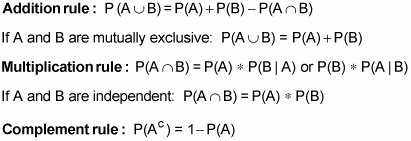## Probability definitions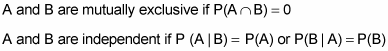## Probability laws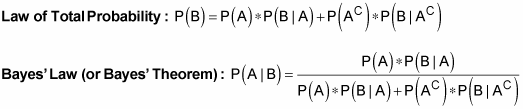## Counting rules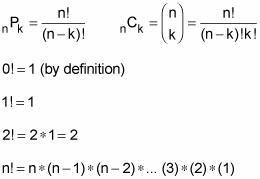## Discrete probability distributions

In probability, a discrete distribution has either a finite or a countably infinite number of possible values. That means you can enumerate or make a listing of all possible values, such as 1, 2, 3, 4, 5, 6 or 1, 2, 3, . . .

There are several kinds of discrete probability distributions, including discrete uniform, binomial, Poisson, geometric, negative binomial, and hypergeometric.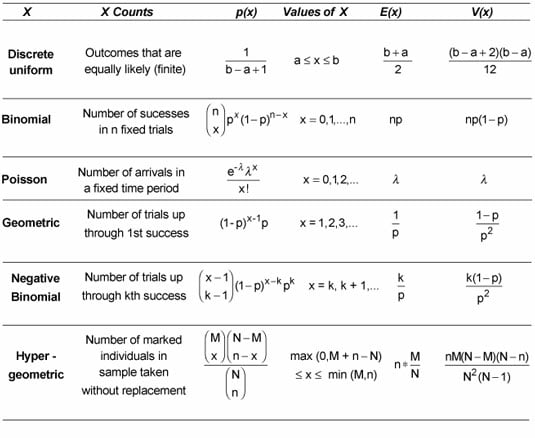## Continuous probability distributions

When you work with continuous probability distributions, the functions can take many forms. These include continuous uniform, exponential, normal, standard normal (Z), binomial approximation, Poisson approximation, and distributions for the sample mean and sample proportion.

When you work with the normal distribution, you need to keep in mind that it’s a continuous distribution, not a discrete one. A continuous distribution’s probability function takes the form of a continuous curve, and its random variable takes on an uncountably infinite number of possible values. This means the set of possible values is written as an interval, such as negative infinity to positive infinity, zero to infinity, or an interval like [0, 10], which represents all real numbers from 0 to 10, including 0 and 10.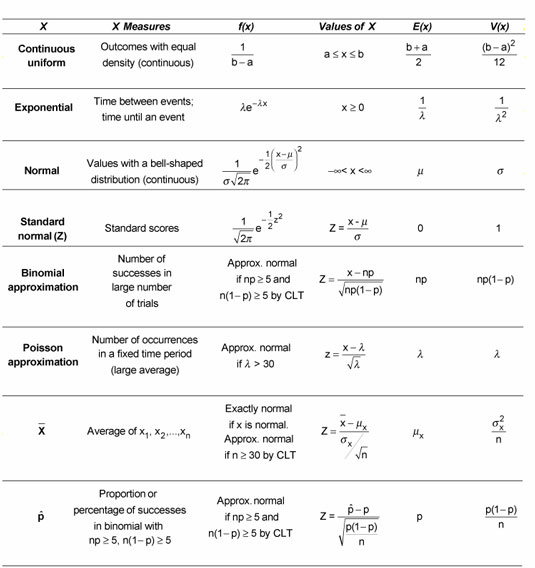## Probability study tips

If you’re going to take a probability exam, you can better your chances of acing the test by studying the following topics. They have a high probability of being on the exam.

• The relationship between mutually exclusive and independent events

• Identifying when a probability is a conditional probability in a word problem

• Probability concepts that go against your intuition

• Marginal, conditional, and joint probabilities for a two-way table

• The Central Limit Theorem: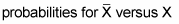• When to use a permutation and when to use a combination

• Finding E(X) from scratch and interpreting it

• Sampling with replacement versus without replacement

• The Law of Total Probability and Bayes’ Theorem

• When the Poisson and exponential are needed in the same problem